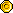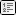### 部落格目录

• Francis S.H的部落格
• 诗词创作
• 无悔的美丽〃• 瓦解〃
• 牵手〃
• 一路上有奶
• 厮守白头〃
• 续梦〃
• 随风往事〃
• 失控的温柔〃
• 幸福的轮廓〃
• 不是奢求〃
• 爱上错过〃
• 精采〃
• 天际〃
• 把握〃
• 幸福滋味〃
• 幸福理由〃
• 来往〃
• 不悔〃
• 这一年那一天〃
• 在我身边〃
• 爱奶想奶〃
• 珍重再见〃
• 孩子气〃
• 逃离心碎〃
• 做好现在〃
• 守候〃
• 永远〃
• 天使的轮廓〃
• 筑梦天堂〃
• 未来〃
• 原来〃
• 永远〃
• 只因为奶〃
• 寒冬〃
• 开始、结束〃
• 在身边〃
• 水平线〃
• 在身边〃
• 一声〃
• 如何是好〃
• 千年之恋〃
• 方向〃
• 恨自己〃
• 等爱〃
• 永夜〃
• 心曲〃
• 续〃
• 付出〃
• 菸〃
• 灵魂〃
• 等奶〃
• 我的爱〃
• 幸福天空〃
• 幸福〃
• 唯情〃
• 放手〃
• 有奶有我〃
• 一无所有〃
• 友谊〃
• 沉默不语〃
• 陪衬〃
• 只是朋友〃
• 相信〃
• 世纪末的锺声〃
• 差距〃
• 结局〃
• 倒影〃
• 话语〃
• 习性〃
• 遇见〃
• 翅膀〃
• 花季〃
• 伸缩自如〃
• 角落〃
• 永不放弃〃
• 旋律〃
• 软弱〃
• 沙漠〃
• 时间比例〃
• 赌局〃
• 这城市〃
• 三个字〃
• 失去〃
• 真心〃
• 我爱奶〃
• 经过〃
• 逃避〃
• 等待〃
• 足够〃
• 永无止境〃
• 期许〃
• 时光〃
• 太平洋〃
• 最远の距离〃
• 我的世界〃
• 感情路〃
• 心灵深处
• 期待能有下一首够爱
• 提醒一下我的生日愿望哦
• 我在拼什麽?
• 将近半年了，属於我们的一切依然记忆犹新...
• 给敬爱的逢森教官• 携手走过，最美的梦。• S.H. 爱写文章
• 使用者所赋与的价值• 惊人的数据，使我更加珍惜。• 没那麽了不起• 人生没有 Total Solution.• IOS 软体推荐
• IOS、Android 免费即时通话软体「Talk Box」• 未分类部落格列表

### QRCode 条码 & APP 连结

说明
本页网址在相关服务标签中，『扫描条码，在行动装置』或是『点击连结按钮，在本装置』中开启网页或是 APP 相关操作。### 访客浏览统计

• 访客人数 : 4,683,174
• 浏览次数 : 4,867,877
• 浏览深度 : 103.9 %
 羊皮纸服务 国际大廰台湾大廰日本大廰对象 说话对谈密语讯息 发送 登入加入会员 分享